# Boylestad: MCQ in DC Biasing – BJTs

(Last Updated On: December 2, 2019)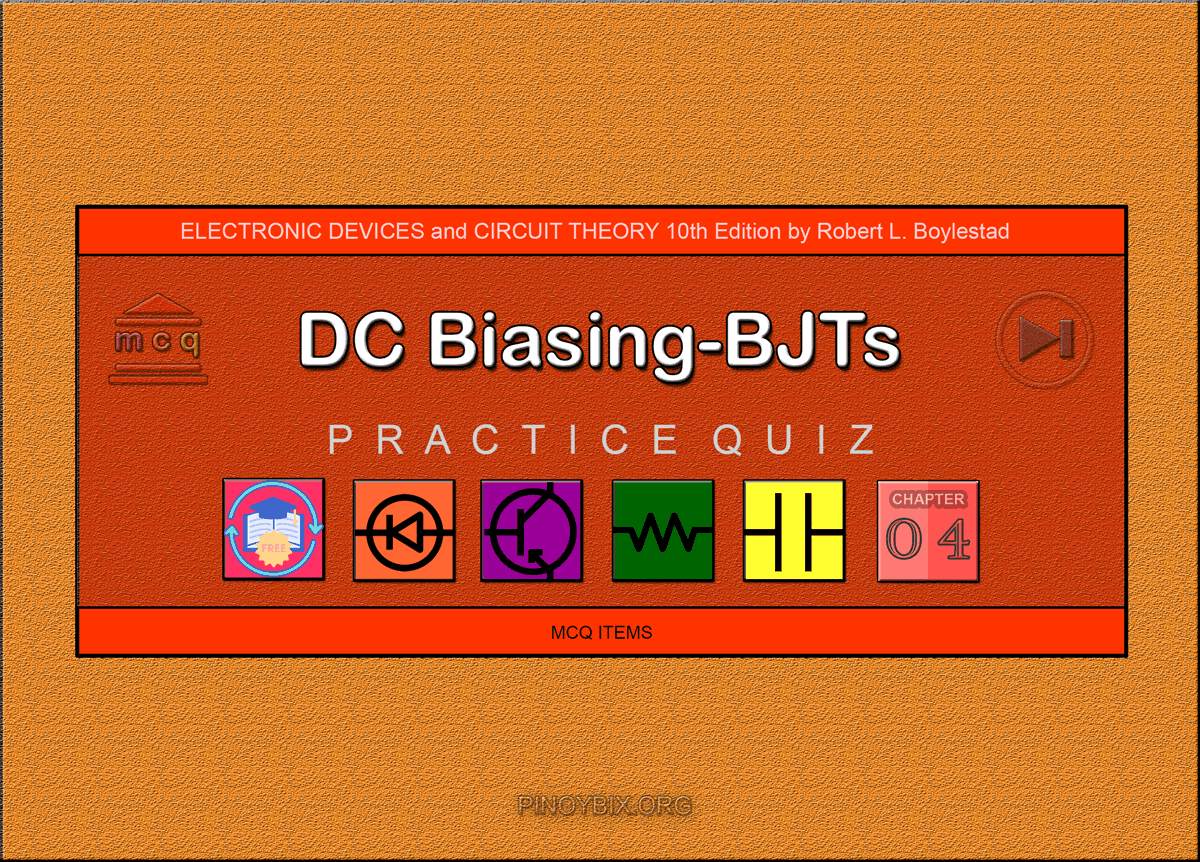This is the Multiple Choice Questions in DC Biasing – BJTs from the book Electronic Devices and Circuit Theory 10th Edition by Robert L. Boylestad. If you are looking for a reviewer in Electronics Engineering this will definitely help. I can assure you that this will be a great help in reviewing the book in preparation for your Board Exam. Make sure to familiarize each and every questions to increase the chance of passing the ECE Board Exam.

#### Online Questions and Answers Topic Outline

• MCQ in Operating Point
• MCQ in Fixed-Bias Circuit
• MCQ in Emitter-Stabilized Bias Circuit
• MCQ in Voltage-Divider Bias
• MCQ in DC Bias with Voltage Feedback
• MCQ in Miscellaneous Bias Configurations
• MCQ in Design Operations
• MCQ in Transistor Switching Networks
• MCQ in Troubleshooting Techniques
• MCQ in PNP Transistors
• MCQ in Bias Stabilization

#### Practice Exam Test Questions

Choose the letter of the best answer in each questions.

1. Which of the following currents is nearly equal to each other?

A) IB and IC

B) IE and IC

C) IB and IE

D) IB, IC, and IE

Solution:

2. The ratio of which two currents is represented by β?

A) IC and IE

B) IC and IB

C) IE and IB

D) None of the above

Solution:

3. At what region of operation is the base-emitter junction forward biased and the base-collector junction reverse biased?

A) Saturation

B) Linear or active

C) Cutoff

D) None of the above

Solution:

4. Calculate the approximate value of the maximum power rating for the transistor represented by the output characteristics of Figure 4.1?

A) 250 mW

B) 170 mW

C) 50 mW

D) 0 mW

Solution:

5. The cutoff region is defined by IB _____ 0 A.

A) >

B) <

C) ≤

D) ≥

Solution:

6. The saturation region is defined by VCE _____ VCEsat.

A) >

B) <

C) ≤

D) ≥

Solution:

7. For the BJT to operate in the active (linear) region, the base-emitter junction must be _______-biased and the base-collector junction must be _______-biased.

A) forward, forward

B) forward, reverse

C) reverse, reverse

D) reverse, forward

Solution:

8. For the BJT to operate in the saturation region, the base-emitter junction must be _______-biased and the base-collector junction must be _______-biased.

A) forward, forward

B) forward, reverse

C) reverse, reverse

D) reverse, forward

Solution:

9. Which of the following voltages must have a negative level (value) in any npn bias circuit?

A) VBE

B) VCE

C) VBC

D) None of the above

Solution:

10. For what value of β does the transistor enter the saturation region?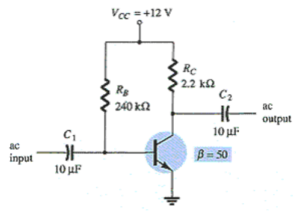A) 20

B) 50

C) 75

D) 116

Solution:

11. Determine the reading on the meter when VCC = 20 V, RC = 5 kΩ, and IC = 2 mA.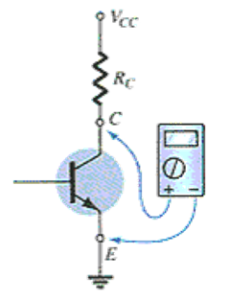A) 10 V

B) –10 V

C) 0.7 V

D) 20 V

Solution:

12. Which of the following is assumed in the approximate analysis of a voltage divider circuit?

A) IB is essentially zero amperes.

B) R1 and R2 are considered to be series elements.

C) βRE ≥ 10R2

D) All of the above

Solution:

13. It is desirable to design a bias circuit that is independent of the transistor beta.

A) True

B) False

Solution:

14. Calculate the voltage across the 91 kΩ resistor.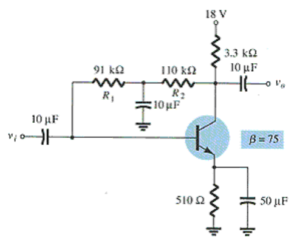A) 18 V

B) 9.22 V

C) 3.23 V

D) None of the above

Solution:

15. Calculate the value of VCEQ.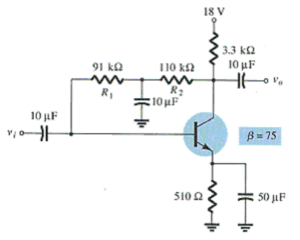A) 8.78 V

B) 0 V

C) 7.86 V

D) 18 V

Solution:

A) 35.29 mA

B) 5.45 mA

C) 1.86 mA

D) 4.72 mA

Solution:

A) 4.52 V

B) –4.52 V

C) 4.48 V

D) –4.48 V

Solution:

A) –4.52 V

B) 4.52 V

C) –9 V

D) 9 V

Solution:

19. Which of the following is (are) related to an emitter-follower configuration?

A) The input and output signals are in phase.

B) The voltage gain is slightly less than 1.

C) Output is drawn from the emitter terminal.

D) All of the above

Solution:

20. Determine the values of VCB and IB for this circuit.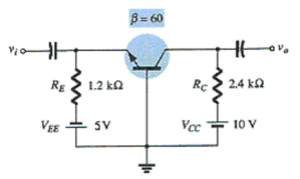A) 1.4 V, 59.7 µA

B) –1.4 V, 59.7 µA

C) –9.3 V, 3.58 µA

D) 9.3 V, 3.58 µA

Solution:

21. Calculate ETh for this network.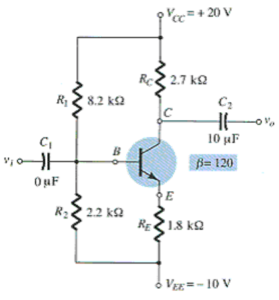A) −12.12 V

B) 16.35 V

C) −3.65 V

D) 10 V

Solution:

22. Calculate Rsat if VCE = 0.3 V.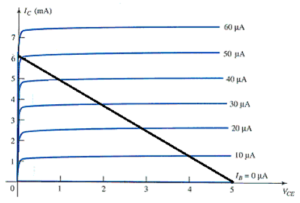A) 49.2 Ω

B) 49.2 kΩ

C) 49.2 mΩ

D) 49.2 MΩ

Solution:

23. You can select the values for the emitter and collector resistors from the information that is provided for this circuit.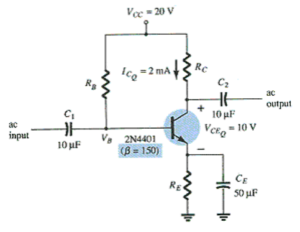A) True

B) False

Solution:

24. In the case of this circuit, you must assume that VE = 0.1VCC in order to calculate RC and RE.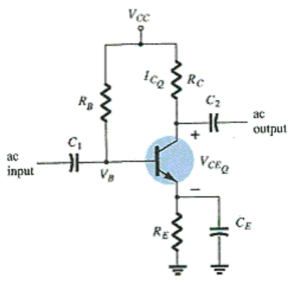A) True

B) False

Solution:

25. Which of the following is (are) the application(s) of a transistor?

A) Amplification of signal

B) Switching and control

C) Computer logic circuitry

D) All of the above

Solution:

26. Calculate the storage time in a transistor switching network if toff is 56 ns, tf = 14 ns, and tr = 20 ns.

A) 70 ns

B) 42 ns

C) 36 ns

D) 34 ns

Solution:

27. The total time required for the transistor to switch from the “off” to the “on” state is designated as ton and defined as the delay time plus the time element.

A) True

B) False

Solution:

28. For an “on” transistor, the voltage VBE should be in the neighborhood of 0.7 V.

A) True

B) False

Solution:

29. For the typical transistor amplifier in the active region, VCE is usually about _____ % to _____ % of VCC.

A) 10, 60

B) 25, 75

C) 40, 90

Solution:

30. Which of the following is (are) a stability factor?

A) S(ICO)

B) S(VBE)

C) S(β)

D) All of the above

Solution:

31. In a fixed-bias circuit, which one of the stability factors overrides the other factors?

A) S(ICO)

B) S(VBE)

C) S(β)

D) Undefined

Solution:

32. In a voltage-divider circuit, which one of the stability factors has the least effect on the device at very high temperature?

A) S(ICO)

B) S(VBE)

C) S(β)

D) Undefined

Solution:

33. Use this table to determine the change in IC from 25°C to 175°C for RB/RE = 250 due to the S(ICO) stability factor. Assume an emitter-bias configuration.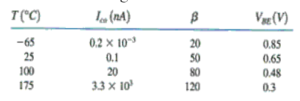A) 140.34 nA

B) 140.34 µA

C) 42.53 nA

D) 0.14034 nA

Solution:

34. Determine the change in IC from 25°C to 175°C for the transistor defined in this table for fixed-bias with RB = 240 kΩ and β = 100 due to the S(VBE) stability factor.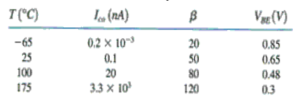A) 145.8 µA

B) 145.8 nA

C) –145.8 µA

D) –145.8 nA

Solution:

35. Determine ICQ at a temperature of 175°C if ICQ = 2 mA at 25°C for RB/RE = 20 due to the S(β) stability factor.

A) 2.417 mA

B) 2.392 mA

C) 2.25 mA

D) 2.58 mA

Solution:

#### Fill-in-the-blanks Questions

1. By definition, quiescent means _______.

A) quiet

B) still

C) inactive

D) All of the above

Solution:

2. ______ should be considered in the analysis or design of any electronic amplifiers.

A) dc

B) ac

C) dc and ac

D) None of the above

Solution:

3. For the dc analysis the network can be isolated from the indicated ac levels by replacing the capacitor with _______.

A) an open circuit equivalent

B) a short circuit equivalent

C) a source voltage

D) None of the above

Solution:

4. In a fixed-bias circuit with a fixed supply voltage VCC’ the selection of a ______ resistor sets the level of ______ current for the operating point.

A) collector, base

B) base, base

C) collector, collector

D) None of the above

Solution:

5. Changes in temperature will affect the level of _______.

A) current gain β

B) leakage current ICEO

C) both current gain β and leakage current ICEO

D) None of the above

Solution:

6. In a fixed-bias circuit, the magnitude of IC is controlled by and therefore is a function of _______.

A) RB

B) RC

C) β

D) RB and β

Solution:

7. For a transistor operating in the saturation region, the collector current IC is at its _______ and the collector-emitter voltage VCE is to the _______.

A) minimum, left of the VCEsat line

B) minimum, right of the VCEsat line

C) maximum, left of the VCEsat line

D) maximum, right of the VCEsat line

Solution:

8. The dc load line is determined solely by the _______.

A) base-emitter loop

B) collector-emitter loop

C) base-collector loop

D) None of the above

Solution:

9. A change in value of _______ will create a new load line parallel to its previous one in a fixed-bias circuit.

A) RB

B) RC

C) VCC

D) VBE

Solution:

10. In a fixed-bias circuit, the slope of the dc load line is controlled by _______.

A) RB

B) RC

C) VCC

D) IB

Solution:

11. The emitter resistor in an emitter-stabilized bias circuit appears to be _______ in the base circuit.

A) larger

B) smaller

C) the same

D) None of the above

Solution:

12. _______ is the primary difference between the exact and approximate techniques used in the analysis of a voltage divider circuit.

A) Thevenin voltage ETh

B) Thevenin resistance RTh

C) Base voltage VB

D) RC

Solution:

13. The Thevenin equivalent network is used in the analysis of the _______ circuit.

A) fixed bias

B) emitter-stabilized bias

C) voltage divider

D) voltage feedback

Solution:

14. The saturation current of a transistor used in a fixed-bias circuit is ________ its value used in an emitter-stabilized or voltage-divider bias circuit for the same values of RC’

A) more than

B) the same as

C) less than

D) None of the above

Solution:

15. In a collector feedback bias circuit, the current through the collector resistor is _______ and the collector current is _______.

A) IC’ IC

B) IB + IC’ IC

C) IB’, IC

D) None of the above

Solution:

16. _______ is the least stabilized circuit.

A) Fixed bias

B) Emitter-stabilized bias

C) Voltage divider

D) Voltage feedback

Solution:

17. _______ is less dependent on the transistor beta.

A) Fixed bias

B) Emitter bias

C) Voltage divider

D) Voltage feedback

Solution:

18. In a transistor-switching network, the level of the resistance between the collector and emitter is _______ at the saturation and is _______ at the cutoff.

A) low, low

B) low, high

C) high, high

D) high, low

Solution:

19. In a transistor-switching network, the operating point switches from _______ to _______ regions along the load line.

A) cutoff, active

B) cutoff, saturation

C) active, saturation

D) None of the above

Solution:

20. For the typical transistor amplifier in the active region, VCE is usually about _____ % to _____ % of VCC’

A) 0, 100

B) 25, 75

C) 45, 55

D) None of the above

Solution:

21. In any amplifier employing a transistor, the collector current IC is sensitive to ______.

A) β

B) VBE

C) ICO

D) All of the above

Solution:

22. As the temperature increases, β ______, VBE ______, and ICO ______ in value for every 10ºC.

A) increases, decreases, doubles

B) decreases, increases, remains the same

C) decreases, increases, doubles

D) increases, increases, triples

Solution:

23. A significant increase in leakage current due to increase in temperature creates _______ between IB curves.

A) smaller spacing

B) larger spacing

C) the same space as at lower temperature

D) None of the above

Solution:

24. The _______ the stability factor, the _______ sensitive the network is to variations in that parameter.

A) higher, more

B) higher, less

C) lower, more

D) None of the above

Solution:

25. In an emitter-bias configuration, the _______ the resistance RE’, the _______ the stability factor, and the _______ stable is the system.

A) smaller, lower, less

B) larger, more, more

C) smaller, more, more

D) larger, lower, more

Solution:

### Complete List of Chapter Quiz in Electronic Devices and Circuit Theory

Help Me Makes a Difference!

 P inoyBIX educates thousands of reviewers/students a day in preparation for their board examinations. Also provides professionals with materials for their lectures and practice exams. Help me go forward with the same spirit. “Will you make a small \$5 gift today?” Option 1 : \$1 USD Option 2 : \$3 USD Option 3 : \$5 USD Option 4 : \$10 USD Option 5 : \$25 USD Option 6 : \$50 USD Option 7 : \$100 USD Option 8 : Other Amount© 2014 PinoyBIX Engineering. © 2019 All Rights Reserved | How to Donate? |#### GEAS Solution

Dynamics problem Economics problem Physics problem Statics problem Strength problem Thermodynamics problem

#### Questions and Answers in GEAS

Engineering Economics Engineering Laws and Ethics Engineering Management Engineering Materials Engineering Mechanics General Chemistry Physics Strength of Materials Thermodynamics
Consider Simple Act of Caring!: LIKE MY FB PAGE

Our app is now available on Google Play, Pinoybix Elex